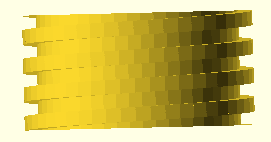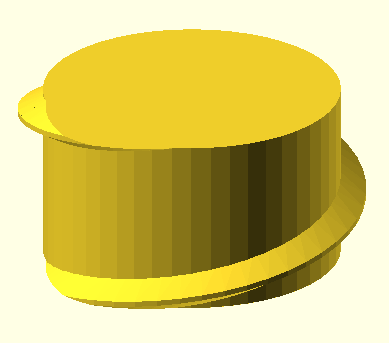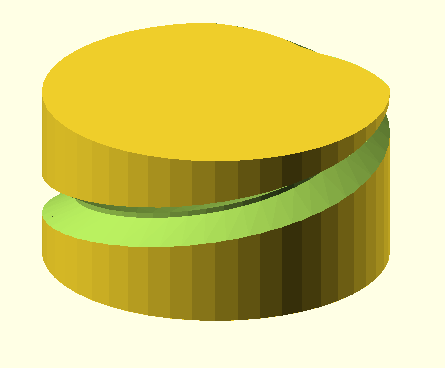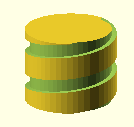Created using this module! By ixd_ui_id Creative Interface Design

Updated 2023-11-20. Ver 2.8; a few speed-ups (thanks to Odino).

Here are modules to draw accurate, ISO-standard threads in OpenSCAD. The specification comes from Wikipedia.

tarball (cd8df981183b9e00f781987b39c05acc)

There are two front-end modules:

metric_thread() input units are millimeters. english_thread() inputs are in inches, though the drawing dimensions are in millimeters.

There are several optional parameters that can be used with either of these modules:

• internal=true. Indicates that an internal thread (e.g., for a nut) will be drawn. Internal threads should be "cut out" from a solid using difference(). The default (internal=false) indicates that external threads (e.g., for a bolt) will be drawn. (The clearances for internal and external threads are different. See Wikipedia.)
• n_starts=1 (default). This provides the option to draw multi-start threads. These have two or more "parallel grooves" for the threads. For example, the DNA double-helix has two "starts". I used this option to create a sprocket for a Rohloff 14-speed bicycle hub, which has a proprietary six-start metric thread.
•square=true. (default: false). Standard square threads (per Wikipedia).

•thread_size=N. (default: same as pitch). Non-standard. This option allows the size of a single thread "V" to be specified independently of the pitch ("travel" per turn). In this example, the pitch is 4 mm, but the thread size is 1 mm.

•groove=true. (default: false) Non-standard. Draws the thread as an inverted "V" subtracted from the "bolt cylinder". In this example, the pitch is 4 mm and the thread size is 1 mm (as in the previous example), but groove is set true.

•rectangle=RATIO. (default: 1). Non-standard. Specifies a "square" thread that is actually a rectangle. In this example, rectangle=0.333 - the "height" of the thread is one-third of its "width." In addition, the pitch is 8 mm while the thread size is 6 mm, and groove is set to true.

• angle=DEG (default: 30). Non-standard. Specifies the angle of the thread side measured from a line perpendicular to the thread axis. The default 30 degrees is the ISO standard.
• taper=diameter/length (default: 0). The amount of taper as change in diameter per length. The NPT standard is 1 inch change in diameter per 16 inches length.
• leadin=N (default: 0). Chamfer end of threads. 0: no chamfer; 1: chamfer at max-z end; 2: chamfer at both ends; 3: chamfer at z=0 end.

Note: left-hand threads. While there is no option for left-hand threads, such "reverse" threads are easy to create in OpenSCAD – use the "mirror" command to "flip" the part across a plane.

This module, which uses a polyhedron for each segment of the thread, was inspired by Trevor Moseley's work, although the results there do not appear to adhere to the ISO standards (especially with regard to required clearances).